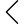52 products found
• s
• m
• l
• xl
PRINTED T-SHIRTS
\$129.00 \$83.85
• s
• m
• l
• xl
• s
• m
• l
• xl
• s
• m
• l
• xl
• s
• m
• l
• xl
• s
• m
• l
• xl
• s
• m
• l
• xl
• s
• m
• l
• xl
• s
• m
• l
• xl
• s
• m
• l
• xl
• s
• m
• l
• xl
• s
• m
• l
• xl
• s
• m
• l
• xl
MODULAR DENIM VEST/COAT
\$409.00 \$265.85
• s
• m
• l
• xl
MODULAR DENIM VEST/COAT
\$409.00 \$265.85
• s
• m
• l
• xl
• s
• m
• l
• xl
• s
• m
• l
• xl
• s
• m
• l
• xl
• s
• m
• l
• xl
• s
• m
• l
• xl
• s
• m
• l
• xl
• s
• m
• l
• xl
• s
• m
• l
• xl
• s
• m
• l
• xl
• s
• m
• l
• xl
• s
• m
• l
• xl
• s
• m
• l
• xl
• s
• m
• l
• xl
• s
• m
• l
• xl
• s
• m
• l
• xl
• s
• m
• l
• xl
• s
• m
• l
• xl
• s
• m
• l
• xl
• s
• m
• l
• xl
• s
• m
• l
• xl
MULTI LAYERED DENIM VEST
\$459.00 \$298.35
• s
• m
• l
• xl
MULTI LAYERED DENIM VEST
\$459.00 \$298.35
• s
• m
• l
• xl
• 2xl
• 3xl
PRINTED T-SHIRTS
\$129.00 \$83.85
• s
• m
• l
• xl
PRINTED T-SHIRTS
\$129.00 \$83.85
•• 1
• 2